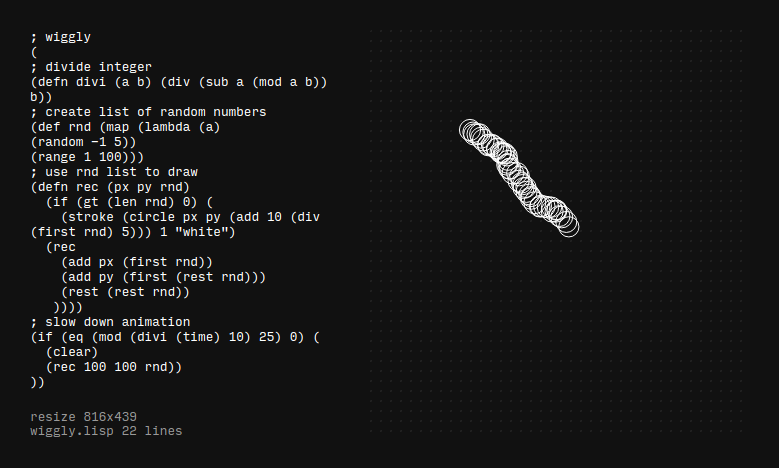## Ronin experiment 4 - wiggly

ronin experiment no 4: a wiggly line created using a recursive lisp function only updating every n milliseconds to slow down the animationThis is the code I used to create the animation

``````; wiggly
(
; divide integer
(defn divi (a b) (div (sub a (mod a b)) b))
; create list of random numbers
(def rnd (map (lambda (a)
(random -1 5))
(range 1 100)))
; use rnd list to draw
(defn rec (px py rnd)
(if (gt (len rnd) 0) (
(stroke (circle px py (add 10 (div (first rnd) 5))) 1 "white")
(rec
(rest (rest rnd))
))))
; slow down animation
(if (eq (mod (divi (time) 10) 25) 0) (
(clear)
(rec 100 100 rnd))
))
``````